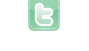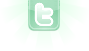# move actors

hello, I am trying to make a game where 15 vertical rectangles (with a hole in the middle ) are moving vertical, each with a different starting time(not sync). I created the actors with a string. So automaticly 15 actors spawn, but how do i make them move seperated so not sycnronised? thnx
int x = Greenfoot.getRandomNumber(getWidth()); int y = Greenfoot.getRandomNumber (getHeight()); Verti verticalebalk= new Verti(); addObject(verticalebalk,300,200); balk balk1 = new balk (); for (int i=0; i<1; i++) { addObject(new balk(),90+i*30,200); }
Tlax wrote...
hello, I am trying to make a game where 15 vertical rectangles (with a hole in the middle ) are moving vertical, each with a different starting time(not sync). I created the actors with a string. So automaticly 15 actors spawn, but how do i make them move seperated so not sycnronised? thnx
Either add them into the world at different times or add a randomized delay timer for the instances of the actor class that creates the wanna-be non-synced actors.
first thnx for the anwser, how can i put a delay timer ? The goal is to have the objects move vertical non-synced and when they are in the screen the player will have to pres space to stop the actor, and this then 15 times.
when they are in the middle of the screen **
Tlax wrote...
```int x = Greenfoot.getRandomNumber(getWidth());
int y = Greenfoot.getRandomNumber (getHeight());
Verti verticalebalk= new Verti();
balk balk1 = new balk ();
for (int i=0; i<1; i++)
{
}```
In code provided, x and y are not used; so lines 1 and 2 can be removed. Also, balk1 is not used, so line 5 can also be removed. The for loop only executed once, so with no real looping, lines 6, 7 and 9 can be removed. That leaves the following:
```addObject(new Verti(), 300, 200);
Obviously, this is deficient for your needs.
Tlax wrote...
The goal is to have the objects move vertical non-synced and when they are in the screen the player will have to pres space to stop the actor, and this then 15 times.
Referring to "when they are in the screen", does this mean that they start off-screen? If so, are you using an unbounded world where actors can actually go off-screen?
how can i put a delay timer ?
You may not need to use a delay timer. You may only have to place the actors randomly off-screen so that there will be no synchronization among them.
```import greenfoot.*;

public class Balk extends Actor
{
static boolean stopped;

int dir = 1-2*Greenfoot.getRandomNumber(2);
int speed = 1; // adjust as needed (always positive)

public void act()
{
if (stopped)
{
// possible code to restart (set stopped to false again)
return;
}
setLocation(getX(), getY()+dir*speed);
int worldHeight = getWorld().getHeight();
int height = getImage().getHeight();
if ((getY() < height/2 && dir == -1) || (getY() > worldHeight-height/2 && dir == 1) dir = -dir;
}
}```
In world:
```for (int i=0; i<15; i++)
{
int y = Greenfoot.getRandomNumber(getHeight());
}```
Player stops Balk objects with:
`Balk.stopped = true;`
With delay timer field, you would have:
```import greenfoot.*;

public class Balk extends Actor
{
static boolean stopped = true;

int dir = 1-2*Greenfoot.getRandomNumber(2);
int speed = 1; // adjust as needed (always positive)
int delay = Greenfoot.getRandomNumber(400/speed);

public void act()
{
if (stopped)
{
delay--;
if (delay == 0) stopped = false;
return;
}
setLocation(getX(), getY()+dir*speed);
int wh = getWorld().getHeight();
int h = getImage().getHeight();
if ((getY() < h/2 && dir == -1) || (getY() > wh-h/2 && dir == 1) dir = -dir;
}
}```
In world:
`for (int i=0; i<15; i++) addObject(new Balk(), 90+30*i, 200);`
thank you very much